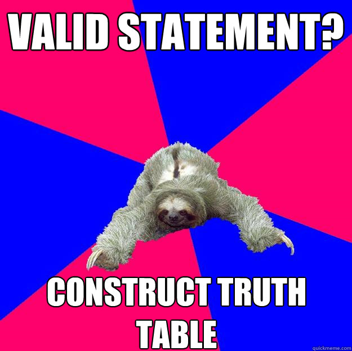Die Logik ist keine Lehre, sondern ein Spiegelbild der Welt.
Logik ist transzendental.

- Ludwig Wittgenstein's (1921, 6.13) Tractatus Logico-Philosophicus in the original German

Logic is not a body of doctrine, but a mirror-reflection of the world.
Logic is transcendental.
- Pears/McGuinness translation

## Truth Table Test

1. Criterion for argumentative validity:
2. Assuming the truth of all the premises, the conclusion set cannot be false
3. (Alternatively) the conclusion set follows from the premises as a matter of logical necessity

4. Criterion for argumentative soundness:
5. CONDITION 1: The argument is valid
6. CONDITION 2: All the premises of the argument are true

#### Truth table for p ⟷ q

 p q p ⟷ q 0 0 1 1 0 0 0 1 0 1 1 1

1. The criterion of argumentative validity relies on the truth values of propositions
2. A truth table represents the truth values of propositions
3. ∴ A truth table test could in principle be constructed to illustrate the validity of an argument

4. CAVEATS:
5. CAVEAT 1: The number of rows increases exponentially as the number of propositional variables increases
6. Assuming classical bivalence and with n propositional variables, we get 2n rows in our truth table

7. CAVEAT 2: Things also get more complicated if we admit more than 2 truth values
8. With a trivalent or 3-valued system of logic and n propositional variables, we get 3n rows in our truth table1. Where φL ψ denotes an argument:
2. STEP 1: Decompose all the constituents of the argument (viz. φ, ψ) into their propositional atoms
3. STEP 2: On the LHS side of the truth table, identify all possible combinations of input truth values for these propositional atoms
4. STEP 3: On the RHS of the truth table, compute all the associated output truth values of the argumentative constituents (i.e. members of φ and ψ)
5. STEP 4: Scan every row of the truth table
6. STEP 5: Identify the rows where the premises (i.e. members of φ) are all true
7. STEP 6: If the conclusion (i.e. members of ψ) is false in at least one of these rows, then the argument is invalid
8. Otherwise, the argument is valid

## P1: p → q P2: ∼q C: ∴ ∼p

EXAMPLE of an argument

#### Truth table test

 LHS (propositional atoms and all possible combinations of input truth values) RHS (associated output truth values of the argumentative premises and conclusion) p q p → q (or P1) ∼q (or P2) ∴ ∼p (or C) 0 0 1 1 1 1 0 0 1 0 0 1 1 0 1 1 1 1 0 0

1. It is only in INTERPRETATION 1 that all the argumentative premises (P1 and P2) are true
2. The output truth value of the conclusion (C) is true
3. ∴ The argument is valid
4. We have just used the truth table test to illustrate the validity of this argument
5. This argument is a valid rule of inference: it is known as the modus tollens rule of inference